# Shunt Resistor: What is it And How Does it Work?

Contents

## What is a Shunt Resistor?

A shunt resistor (or shunt) is defined as a device that creates a low resistance path to force most of the electric current through the circuit to flow through this path. In most cases, a shunt resistor is made up of a material having a low-temperature coefficient of resistance, giving it a very low resistance over a wide temperature range.

Shunt resistors are commonly used in current measuring devices called “ammeters”. In an ammeter, the shunt resistance is connected in parallel. An ammeter is connected in series with a device or circuit.

## How Does a Shunt Resistor Work?

A shunt resistor has a low resistance. It provides a low resistance path to current, and it is connected in parallel with a current measuring device.

The shunt resistor uses ohm’s law to measure the current. The resistance of the shunt resistor is known. And it is connected in parallel with the ammeter. So, the voltage is the same.

Hence, if we measure the voltage across a shunt resistance, we can measure the current passing through the device by the equation below of ohm’s law.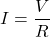## Using a Shunt Resistor to Measure Current

Consider an ammeter that has resistance Ra and measures a very small current Ia. For exceeding the range of an ammeter, a shunt resistor Rs is placed parallel with Rm.

The circuit diagram of these connections is shown in the figure below.

The total current supplied by the source is I. It is divided into two paths.

According to Kirchhoff’s current law (KCL),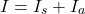Where,

Is = current passes through resistance Rs (shunt current)

Ia = current passes through resistance Ra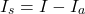The shunt resister Rs is connected parallel with resistor Ra. Hence the voltage drop across both resisters are equal.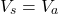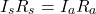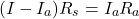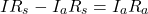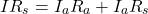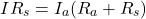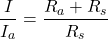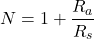Where N is a multiplying power of shunt.

## How to Build a Shunt Resistor

The shunt resister can be built with very thin copper wires. The length and size of the shunt resister depend on the resistance you require.

The resistance of the shunt resistor will decide the range of the ammeter.

If you use 10 AWG (2.59 mm diameter) copper wire, the resistance of this wire is 0.9989 ohms per 1000 feet. This resistance may vary according to the quality of a wire.

Hence, make sure of the resistance before using it. To calculate the required length of wire for a particular value of shunt resistor, use the below equation.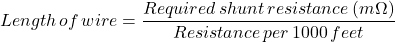For example, if you require a shunt of 0.5 mΩ and use 10 AWG wire, put the above values in this equation.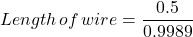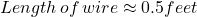## What Is the Purpose of Shunt Resistor (Applications)?

The application of shunt resister is as listed below.

• A shunt resistor is used in an electrical circuit to protect against overvoltage.
• It is used in a current measuring device.
• It is used to bypass defective devices. (When a number of components are connected in series, one component is failed to operate, it will break the entire circuit. In this condition, the shunt resistor bypasses the fail component and continues the circuit path.)
• A shunt resistor with a capacitor is used in a circuit to avoid the problem of high-frequency noise.
• It is used in overload protection control circuits, including power supplies.

## How to Size a Shunt Resistor

Generally, a shunt resistor is used in the current measuring device. The size of the shunt resister will decide the range of the ammeter.

The size of the shunt resister depends on the maximum current rating. It is calculated by the voltage drop at the maximum current rating.

Let say a shunt resistor is designed for maximum current 100 A and voltage drop 50 mV. Typically, the voltage drop at maximum current is 50 mV, 75 mV, or 100 mV.

In this example, we can calculate the resistance of shunt resister by ohm’s law.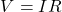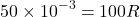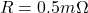Want To Learn Faster? 🎓
Get electrical articles delivered to your inbox every week.
No credit card required—it’s 100% free.